1. /
2. NCERT Solutions
3. /
4. NCERT Solutions for Class...

# NCERT Solutions for Class 9 Maths Exercise 1.4### myCBSEguide App

Download the app to get CBSE Sample Papers 2023-24, NCERT Solutions (Revised), Most Important Questions, Previous Year Question Bank, Mock Tests, and Detailed Notes.

NCERT solutions for Class 9 Maths Number Systems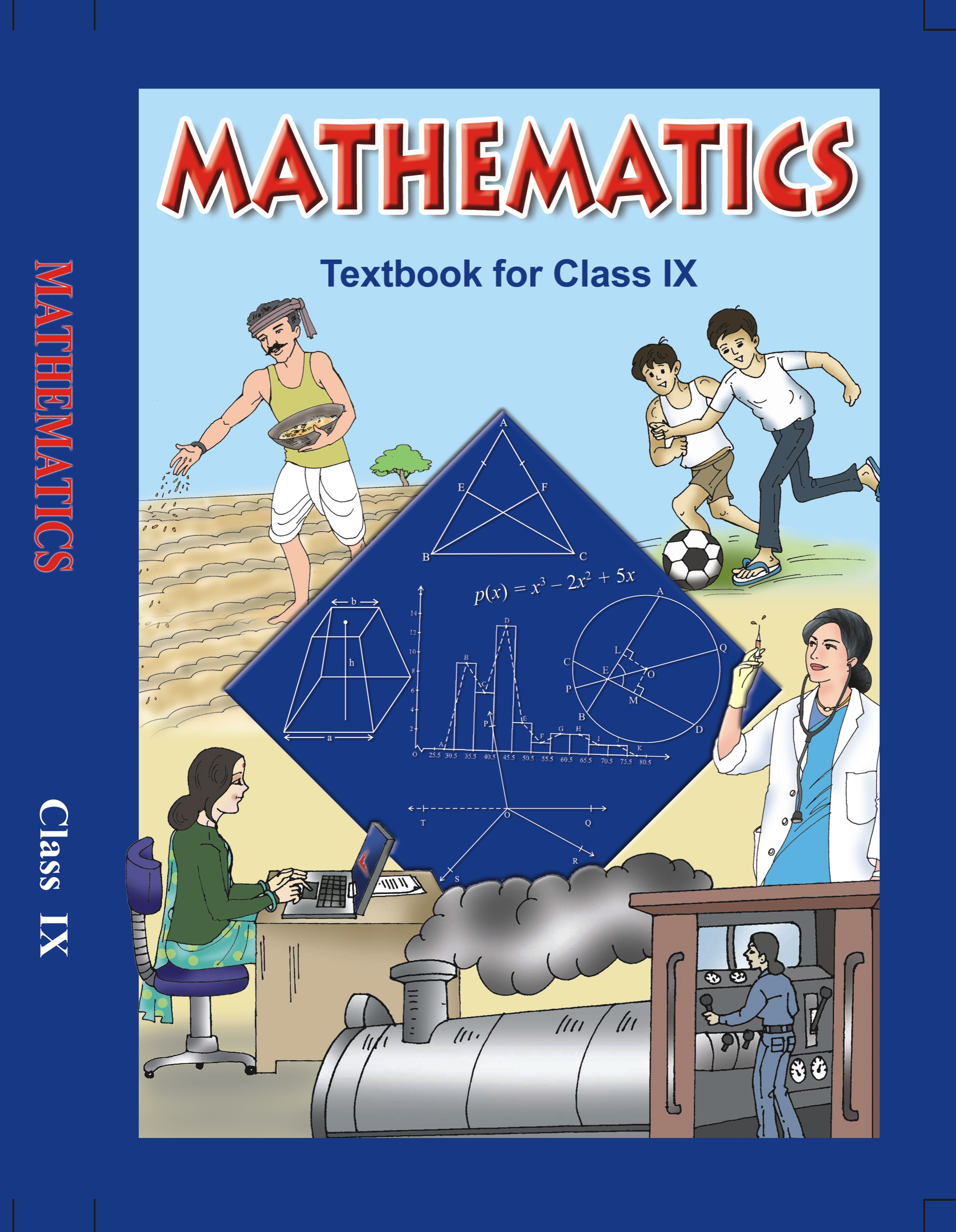## NCERT Solutions for Class 9 Maths Number Systems

###### 1. Visualize 3.765 on the number line using successive magnification.

Ans. We know that the number 3.765 will lie between 3.764 and 3.766.

We know that the numbers 3.764 and 3.766 will lie between 3.76 and 3.77.

We know that the numbers 3.76 and 3.77 will lie between 3.7 and 3.8.

We know that the numbers 3.7 and 3.8 will lie between 3 and 4.

Therefore, we can conclude that we need to use the successive magnification, after locating numbers 3 and 4 on the number line.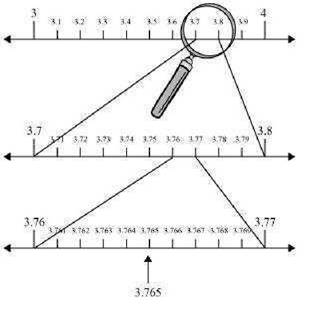###### 2. Visualize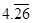on the number line, up to 4 decimal places.

Ans. We know that the numbercan also be written as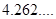.

We know that the numberwill lie between 4.261 and 4.263.

We know that the numbers 4.261 and 4.263 will lie between 4.26 and 4.27.

We know that the numbers 4.26 and 4.27 will lie between 4.2 and 4.3.

We know that the numbers 4.2 and 4.3 will lie between 4 and 5.

Therefore, we can conclude that we need to use the successive magnification, after locating numbers 4 and 5 on the number line.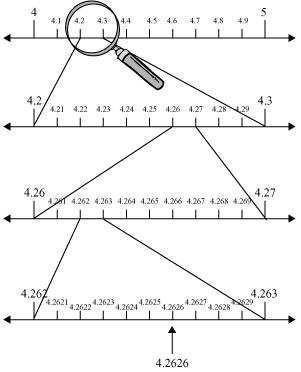## NCERT Solutions for Class 9 Maths Exercise 1.4

NCERT Solutions Class 9 Maths PDF (Download) Free from myCBSEguide app and myCBSEguide website. Ncert solution class 9 Maths includes text book solutions from Mathematics Book. NCERT Solutions for CBSE Class 9 Maths have total 15 chapters. 9 Maths NCERT Solutions in PDF for free Download on our website. Ncert Maths class 9 solutions PDF and Maths ncert class 9 PDF solutions with latest modifications and as per the latest CBSE syllabus are only available in myCBSEguide.

## CBSE app for Class 9

To download NCERT Solutions for Class 9 Maths, Computer Science, Home Science,Hindi ,English, Social Science do check myCBSEguide app or website. myCBSEguide provides sample papers with solution, test papers for chapter-wise practice, NCERT solutions, NCERT Exemplar solutions, quick revision notes for ready reference, CBSE guess papers and CBSE important question papers. Sample Paper all are made available through the best app for CBSE students and myCBSEguide website.Test Generator

Create question paper PDF and online tests with your own name & logo in minutes.myCBSEguide

Question Bank, Mock Tests, Exam Papers, NCERT Solutions, Sample Papers, Notes

### 1 thought on “NCERT Solutions for Class 9 Maths Exercise 1.4”

1. This is a very useful website ????